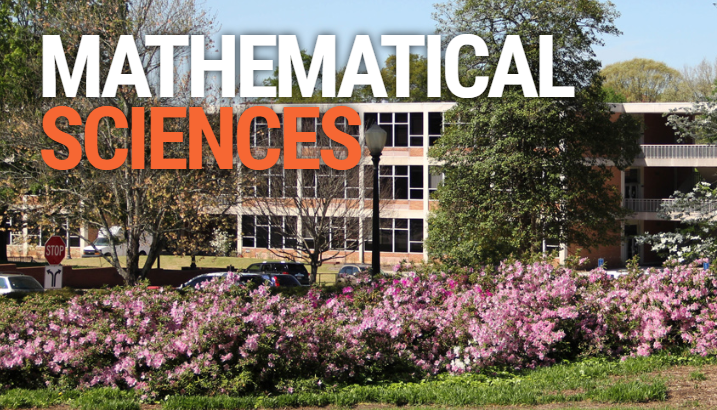# Clemson University, School of Mathematical and Statistical SciencesThe mission of the Department of Mathematical Sciences is to create and discover new knowledge in the mathematical sciences, to disseminate new and existing knowledge in the mathematical sciences, and to apply new and existing knowledge in the mathematical sciences to benefit the economic future of the state and nation.

Mathematical Science is a science in search of pattern, form and structure, whose operating sphere is one of numbers, algorithms, ideas and models. Often using experimentation and simulation it relies on the rigor of logic as its ultimate truth. The term mathematical sciences refers to several interrelated areas of study which are intrinsically mathematical:

• algebra and discrete mathematics,
• analysis and dynamical systems,
• applied and computational mathematics,
• probability and statistics, and
• operations research.

Although core mathematics–with its rich tradition of abstraction, logic, and proof–is the foundation, mathematical sciences also deals with experimentation and data, and employs a variety of mathematical models for the analysis of both deterministic and probabilistic situations.

Clemson's School of Mathematical and Statistical Sciences was among the first in the country to effectively combine the areas of algebra, analysis, computation, statistics, and operations research into a single mathematical sciences program. The result is a balanced, flexible, and modern curriculum which has attracted considerable national attention.